Ask your WordPress questions! Pay money and get answers fast!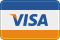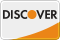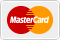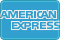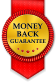# Return query as xml WordPress

• SOLVED

I would like to return this query as xml:

```function return_xml_test( \$attr, \$content ) { global \$wpdb; \$query = \$wpdb->prepare( " SELECT L.cage_desc, I.item_desc, SUM(T.quantity) as sum FROM wp_transactions T, wp_location L, wp_items I WHERE T.item_id = I.item_id AND T.location_id = L.location_id AND T.trans_type= 'Inventory' " ); \$results = \$wpdb->get_results( \$query ); \$return = ''; foreach( \$results as \$result ) { \$return .= \$result->cage_desc . \$result->item_desc . \$result->sum . "</br>\n"; } return \$return; } // Register a new shortcode add_shortcode( '103', 'return_xml_test' );```

Please rewrite the function so that it returns in this format:
```<?xml version="1.0"?> <dataset> <row> <cage_desc></cage_desc> <item_desc></item_desc> <sum></sum> </row> </dataset>```

2011-09-22

``` function return_xml_test( \$attr, \$content ) { global \$wpdb; \$query = \$wpdb->prepare( "SELECT L.cage_desc, I.item_desc, SUM(T.quantity) as sum FROM wp_transactions T, wp_location L, wp_items I WHERE T.item_id = I.item_id AND T.location_id = L.location_id AND T.trans_type= 'Inventory' " ); \$results = \$wpdb->get_results( \$query ); \$format = "<row> <cage_desc>%s</cage_desc> <item_desc>%s</item_desc> <sum>%s</sum> </row>"; \$return = '<?xml version="1.0"?><dataset>'; foreach (\$results as \$result) { \$return .= sprintf(\$format, \$result->cage_desc, \$result->item_desc, \$result->sum); } return \$return .= '</dataset>'; } // Register a new shortcode add_shortcode( '103', 'return_xml_test' ); ```

Luis,
Thanks for replying. Again, the data is returned as a long run on:
1 itemA 992 itemB 993 itemC 994 itemD 99

``` <dataset> <row> <cage_desc>1</cage_desc> <item_desc>itemA</item_desc> <sum>99</sum> </row> <row> <cage_desc>2</cage_desc> <item_desc>itemB</item_desc> <sum>99</sum> </dataset> ```
(etc)

I think it needs to be something like:

print "<dataset>\n";
print " <row>\n";
print " <cage_desc>".\$result->cage_desc." </cage_desc>\n";
print " <item_desc>".\$result->item_desc." </item_desc>\n";
print " <sum>".\$result->sum."</sum>\n";
print " </row>\n";
print "</dataset>\n";

but I don't know how to do that.

any ideas?

Try this
``` function return_xml_test( \$attr, \$content ) { global \$wpdb; \$query = \$wpdb->prepare( "SELECT L.cage_desc, I.item_desc, SUM(T.quantity) as sum FROM wp_transactions T, wp_location L, wp_items I WHERE T.item_id = I.item_id AND T.location_id = L.location_id AND T.trans_type= 'Inventory' " ); \$results = \$wpdb->get_results( \$query ); \$format = "<row> <cage_desc>%s</cage_desc> <item_desc>%s</item_desc> <sum>%s</sum> </row>"; \$return = '<pre><?xml version="1.0"?><dataset>'; foreach (\$results as \$result) { \$return .= sprintf(\$format, \$result->cage_desc, \$result->item_desc, \$result->sum); } return \$return .= '</dataset></pre>'; } // Register a new shortcode add_shortcode( '103', 'return_xml_test' ); ```

Try this, i looks like a better solution
``` function return_xml_test(\$attr, \$content) { global \$wpdb; \$sql = "SELECT L.cage_desc, I.item_desc, SUM(T.quantity) as sum FROM wp_transactions T, wp_location L, wp_items I WHERE T.item_id = I.item_id AND T.location_id = L.location_id AND T.trans_type= 'Inventory' "; \$query = \$wpdb -> prepare(\$sql); \$results = \$wpdb -> get_results(\$query); \$format = "\n\t<row>\n\t<cage_desc>%s</cage_desc>\n\t<item_desc>%s</item_desc>\n\t<sum>%s</sum>\n\t</row>\n"; \$return = "<?xml version=\"1.0\"?>\n<dataset>"; foreach (\$results as \$result) { \$return .= sprintf(\$format, \$result->cage_desc, \$result->item_desc, \$result->sum); } \$return .= '</dataset>'; return '<pre>' . htmlspecialchars(\$return) . '</pre>'; } // Register a new shortcode add_shortcode( '103', 'return_xml_test' ); ```

I added some extra html tags to work with the plugin above.

``` function return_xml_test(\$attr, \$content) { global \$wpdb; \$sql = "SELECT L.cage_desc, I.item_desc, SUM(T.quantity) as sum FROM wp_transactions T, wp_location L, wp_items I WHERE T.item_id = I.item_id AND T.location_id = L.location_id AND T.trans_type= 'Inventory' "; \$query = \$wpdb -> prepare(\$sql); \$results = \$wpdb -> get_results(\$query); \$format = "\n\t<row>\n\t<cage_desc>%s</cage_desc>\n\t<item_desc>%s</item_desc>\n\t<sum>%s</sum>\n\t</row>\n"; \$return = "<?xml version=\"1.0\"?>\n<dataset>"; foreach (\$results as \$result) { \$return .= sprintf(\$format, \$result->cage_desc, \$result->item_desc, \$result->sum); } \$return .= '</dataset>'; return '<pre name="code" class="html">' . htmlspecialchars(\$return) . '</pre>'; } // Register a new shortcode add_shortcode( '103', 'return_xml_test' ); ```

Luis,
Hi, I'm writing the functions for a plugin (private) that uses MySQL data to create a PDF. The data needs to be stored as an XML, prior to creating the PDF. No one will ever actually see the XML file.
The data is never viewed as HTML, but thanks for looking around for me, that was very kind.

The solution you offered
``` \$format = "<row> <cage_desc>%s</cage_desc> <item_desc>%s</item_desc> <sum>%s</sum> </row>"; ```
is the closest to working so far, but it still doesn't print the hierarchy, just the values.
Because I'm creating an XML file, I need it to actually print the hierarchy
``` print "<dataset>\n"; print " <row>\n"; print " <cage_desc>".\$result->cage_desc." </cage_desc>\n"; print " <item_desc>".\$result->item_desc." </item_desc>\n"; print " <sum>".\$result->sum."</sum>\n"; print " </row>\n"; print "</dataset>\n"; ```

Does that make sense?

OK, if you are not displaying in HTML, it should work without the pre tag, i added the <pre> to show the xml as part of the post content.

If you need just the XML code, you can not see it on the HTML, but you can check the page source and you'll see that its OK the structure.

You will see a code like this in "View page source":
``` <?xml version="1.0"?> <dataset> <row> <cage_desc>1</cage_desc> <item_desc>Item A</item_desc> <sum>99</sum> </row> <row> <cage_desc>2</cage_desc> <item_desc>Item B</item_desc> <sum>100</sum> </row> </dataset> ```

Use this:
``` function return_xml_test(\$attr, \$content) { global \$wpdb; \$sql = "SELECT L.cage_desc, I.item_desc, SUM(T.quantity) as sum FROM wp_transactions T, wp_location L, wp_items I WHERE T.item_id = I.item_id AND T.location_id = L.location_id AND T.trans_type= 'Inventory' "; \$query = \$wpdb -> prepare(\$sql); \$results = \$wpdb -> get_results(\$query); \$format = "\n\t<row>\n\t<cage_desc>%s</cage_desc>\n\t<item_desc>%s</item_desc>\n\t<sum>%s</sum>\n\t</row>\n"; \$return = "<?xml version=\"1.0\"?>\n<dataset>"; foreach (\$results as \$result) { \$return .= sprintf(\$format, \$result->cage_desc, \$result->item_desc, \$result->sum); } \$return .= '</dataset>'; return \$return; } // Register a new shortcode add_shortcode( '103', 'return_xml_test' ); ```

Luis,
Oh my gosh, I am NOT the sharpest pencil in the box tonight. :)

It's perfect in view source.

Sorry for the extra work, I appreciate your help!

2011-09-22

``` function return_xml_test( \$attr, \$content ) { global \$wpdb; \$query = \$wpdb->prepare( " SELECT L.cage_desc, I.item_desc, SUM(T.quantity) as sum FROM wp_transactions T, wp_location L, wp_items I WHERE T.item_id = I.item_id AND T.location_id = L.location_id AND T.trans_type= 'Inventory' " ); \$results = \$wpdb->get_results( \$query ); \$return = "<?xml version='1.0' ?>"; foreach( \$results as \$result ) { \$return .="<dataset> <row> <cage_desc>".\$result->cage_desc." </cage_desc> <item_desc>".\$result->item_desc." </item_desc> <sum>".\$result->sum." </sum> </row> </dataset>"; } return \$return; } // Register a new shortcode add_shortcode( '103', 'return_xml_test' ); ```

Romel,
Thanks for replying. The data is returned as a long run on:
1 itemA 992 itemB 993 itemC 994 itemD 99

``` <dataset> <row> <cage_desc>1</cage_desc> <item_desc>itemA</item_desc> <sum>99</sum> </row> <row> <cage_desc>2</cage_desc> <item_desc>itemB</item_desc> <sum>99</sum> </dataset> ```
(etc)

I think it needs to be something like:
``` print "<dataset>\n"; print " <row>\n"; print " <cage_desc>".\$result->cage_desc." </cage_desc>\n"; print " <item_desc>".\$result->item_desc." </item_desc>\n"; print " <sum>".\$result->sum."</sum>\n"; print " </row>\n"; print "</dataset>\n"; ```
but I don't know how to do that.

any ideas?

try this one..i think the double qoutes is making the long result..

```function return_xml_test( \$attr, \$content ) { global \$wpdb; \$query = \$wpdb->prepare( " SELECT L.cage_desc, I.item_desc, SUM(T.quantity) as sum FROM wp_transactions T, wp_location L, wp_items I WHERE T.item_id = I.item_id AND T.location_id = L.location_id AND T.trans_type= 'Inventory' " ); \$results = \$wpdb->get_results( \$query ); \$return = '<?xml version="1.0" ?>'; foreach( \$results as \$result ) { \$return .='<dataset><br/> <row><br/> <cage_desc>'.\$result->cage_desc.' </cage_desc><br/> <item_desc>'.\$result->item_desc.' </item_desc><br/> <sum>'.\$result->sum. </sum><br/> </row><br/> </dataset><br/>'; } return \$return; } // Register a new shortcode add_shortcode( '103', 'return_xml_test' ); ```

and I think you also need to include the header for xml

`header("Content-Type: application/xml");`

by the way is this for an rss?
or you just want the data be displayed in a page or something?

Crashed the server. ;(

I'm writing the functions for a plugin that creates a PDF from MYSQL data. The data needs to be stored as XML before creating the PDF.

ok. try this one up.

``` function return_xml_test( \$attr, \$content ) { global \$wpdb; \$query = \$wpdb->prepare( " SELECT L.cage_desc, I.item_desc, SUM(T.quantity) as sum FROM wp_transactions T, wp_location L, wp_items I WHERE T.item_id = I.item_id AND T.location_id = L.location_id AND T.trans_type= 'Inventory' " ); \$results = \$wpdb->get_results( \$query ); @header('Content-type: text/xml; charset=' . get_option('blog_charset')); \$return = '<?xml version="1.0" encoding="'.get_option('blog_charset').'" standalone="yes"?>'; foreach( \$results as \$result ) { \$return .='<dataset><br/> <row><br/> <cage_desc>'.\$result->cage_desc.' </cage_desc><br/> <item_desc>'.\$result->item_desc.' </item_desc><br/> <sum>'.\$result->sum. </sum><br/> </row><br/> </dataset><br/>'; } return \$return; } // Register a new shortcode add_shortcode( '103', 'return_xml_test' ); ```

2011-09-22

Hi,

you can try this :

``` function return_xml_test( \$attr, \$content ) { global \$wpdb; \$query = \$wpdb->prepare( " SELECT L.cage_desc, I.item_desc, SUM(T.quantity) as sum FROM wp_transactions T, wp_location L, wp_items I WHERE T.item_id = I.item_id AND T.location_id = L.location_id AND T.trans_type= 'Inventory' " ); \$results = \$wpdb->get_results( \$query ); \$return = "<?xml version='1.0' ?>"; \$return .="<dataset>"; foreach( \$results as \$result ) { \$return .="<row>"; \$return .="<cage_desc>".\$result->cage_desc." </cage_desc>"; \$return .="<item_desc>".\$result->item_desc." </item_desc>"; \$return .="<sum>".\$result->sum."</sum>"; \$return .="</row>"; } \$return .= "</dataset>"; return \$return; } // Register a new shortcode add_shortcode( '103', 'return_xml_test' ); ```

Luthfi,
Thanks for replying. The data is returned as a long run on:
1 itemA 992 itemB 993 itemC 994 itemD 99

``` <dataset> <row> <cage_desc>1</cage_desc> <item_desc>itemA</item_desc> <sum>99</sum> </row> <row> <cage_desc>2</cage_desc> <item_desc>itemB</item_desc> <sum>99</sum> </dataset> ```
(etc)

I think it needs to be something like:

print "<dataset>\n";
print " <row>\n";
print " <cage_desc>".\$result->cage_desc." </cage_desc>\n";
print " <item_desc>".\$result->item_desc." </item_desc>\n";
print " <sum>".\$result->sum."</sum>\n";
print " </row>\n";
print "</dataset>\n";

but I don't know how to do that.

any ideas?